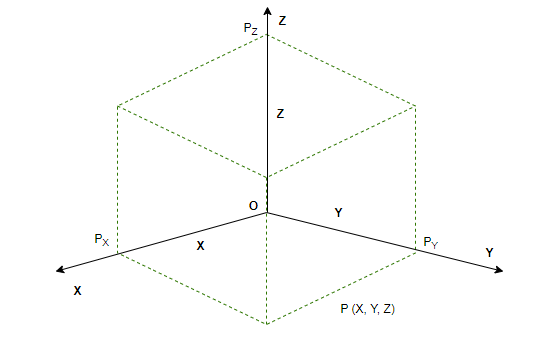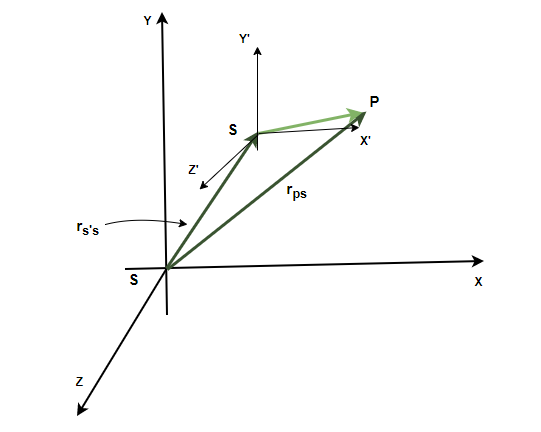# Motion in Three Dimensions

• Last Updated : 20 Nov, 2021

A particle moving in a 1-dimensional space requires only one coordinate to specify its position. In two dimensions similarly, two coordinates are required. Three-dimensional motions are encountered at a lot of places in real life, to analyze these real-life situations. One needs to understand the motion and how to deal with these three coordinates mathematically to describe the trajectories of objects traveling in a three-dimensional plane. Let’s look at these concepts in detail.

### Motion in a Three-Dimensional Space

Suppose a particle is moving between two points in a three-dimensional space. To describe the position of this particle, a position vector is required. These vectors are always with respect to the reference frame at the origin. The following parameters are required to fully describe the behavior of a particle moving in a plane,

1. Position
2. Velocity
3. Acceleration

Position Vector

In a 3-d space, a particle can be anywhere, it cannot be described by just one coordinate. In this case, it is denoted with respect to the origin and it also constitutes the direction one should go into to find that point. That is why a vector is required to describe the position. The vector which denotes the position and direction of the particle’s position with respect to the origin is called the position vector. The position vectorfor a particle is given by,Where x,  y, and z are their components along the x, y, and z-axis.Velocity

The velocity of a particle traveling in the three-dimensional space can be described in two ways – average velocity and instantaneous velocity. When the particle is under acceleration, it changes its velocity every second. So, a single value cannot be assigned to a velocity. In such cases, the instantaneous velocity is preferred, it describes the velocity and its direction at a particular instant. It is given by,Velocity can also be expressed as,The average velocity is the ratio of total displacement over total time. Suppose a particle goes fromtoin a total time ofth="30" style="vertical-align: 0px;"/>

The velocity is given by,Acceleration

The acceleration of a body moving in a plane is given by the rate of change in its velocity. Similar to velocity, there can be two cases here too –  average acceleration and instantaneous acceleration. Average acceleration is given by the ratio of the net change in the velocity of the object with the total time taken. Let initial and final velocities be denoted byandInstantaneous acceleration is used when the acceleration of the body is changing with time.Decomposing this into its components,The magnitude of acceleration can also be calculat

sing the components of velocity,### Relative Motion in Three Dimensions

Relative motion represents the velocity of some body as seen from one frame of reference. These concepts have been known in form of one-dimensional and second-dimensional spaces. But these concepts can be extended to three-dimensional spaces also. Given in the figure below, consider a particle P and reference frames S and S’. The position of the frame S’ as measured in S is rS’S, the position of the particle P as measured with respect to the frame S’ is given by rPS’ and the position of the particle P with respect to the frame of reference S is given by rPS,Notice from the figure that,

rPS  = rPS’  + rS’S

These vectors give us the formula for relative velocities too, differentiating the above equation,Intuitively speaking, the velocity of a particle with respect to S is equal to the velocity of S’ with respect to S plus the velocity of the particle with respect to S. Differentiating this equation again, the equation for the acceleration is given by,="291" style="vertical-align: -9px;"/>The acceleration of a particle with respect to S is equal to the acceleration of S’ with respect to S plus the acceleration of the particle with respect to S.

### Sample Problems

Question 1: Find the velocity at t = 2, for the particle which is moving in a plane and whose position is given, r = t2i + t2j + tk

Given: the initial and final position vectors,

r = t2i + t2j + tk

The velocity in this case is given by the formula,Here x(t) = t2 and y(t) = t2 and z(t) = t

Plug

these values into the equation,At t = 2,Question 2: Find the velocity at t = 0, for the particle which is moving in a plane and whose position is given, r = (t+2)i + (4t2+2)j + t2k

Given: the initial and final position vectors,

r =  (t+2)i + (4t2+2)j + t2k

The velocity in this case is given by the formula,Here x(t) = (t + 2) and y(t) =

up>2 + 2and z(t) = t2

Plugging these values into the equation,At t = 0,⇒ v = i

Question 3: Find the average acceleration between t = 0 and t = 3, for the particle which is moving in a plane and whose position is given, v = 3ti + 3t3j + k

Given: velocity as a function of time.&

;

v = 3ti + 3t3j + k

The velocity vector changes with time. The average acceleration is given by the formula,At t = 0

vi = 0i + 0j  + k

At t = 3

vf = 9i + 81j  + kPlugging the values into the above equation,Question 4: Find the average acceleration between t = 0 and t = 2, for the particle which is moving in a plane and whose pos

n is given, v = ti + 3tj + 2k

Given: velocity as a function of time.

v = ti + 3tj + 2k

The velocity vector changes with time. The average acceleration is given by the formula,At t = 0

vi = 0i + 0j  + 2k

At t = 2

vf = 2i + 6j  + 2kth="78" style="vertical-align: 0px;"/>

Plugging the values into the above equation,Question 5: Find the instantaneous acceleration at t = 1, for the particle which is moving in a plane and whose position is given, v = 2ti + 5t3j + tk

Given: velocity as a function of time.

v = 2ti + 5t3j + tk

The velocity vector changes with time. The instantaneous acceleration is given by the formula,⇒at t = 1,

⇒⇒My Personal Notes arrow_drop_up